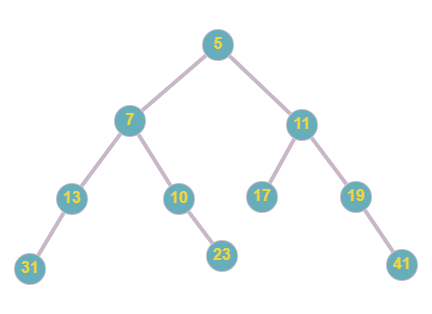# Print all Prime Levels of a Binary Tree

## Introduction

This article discusses how to print all prime levels of a binary tree. A Binary Tree is a special tree data structure where each node has two child nodes only. Such problems may be asked in the coding interviews of many tech companies. Reading this article will surely help you better understand the concepts of Binary Trees and Tree Traversal.

In the given problem, we will perform a level order traversal, check if all the elements of a level are prime or not. If all the elements are prime, we will print the whole level.

## Problem Statement

Given a Binary Tree, print all the prime levels of the tree. That is, print those levels which have all prime numbers as nodes.

## Example

Let us consider this Binary Tree.

Sample_INPUT:Desired_OUTPUT:

5

7 11

31 23 41

## Approach and Explanation

After seeing the above example, it must be clear what we are supposed to do. To clarify the problem again, we will first perform Level Order traversal, then check the nodes for prime; if prime, print that level. In this article, the implementation is done in Java.

To perform the required tasks, the step-by-step approach is as follows:

• Since we are dealing with Binary Tree, we will create it by ourselves. So, we create a constructor TreeNode, with three members- data(int), left(TreeNode), and right(TreeNode).

• Inside the constructor, we take the data of the node and store it. We also initialize the left and right pointers to null.

• We declare a function that takes the user inputted binary tree and performs a level order traversal. The level order traversal is saved in a queue. Let us call this function printPrimeTreeLevels(). From this function, we will call another function, say calcPrimeLvl().

• In calcPrimeLvl(), we pass the queue, the start index, and the size.
• This function is responsible for getting the prime level of the given Binary Tree. We check if the root is prime or not using checkPrime() function.
• We traverse one by one to check if the elements of the level are prime or not.
• To ensure the given level is all prime or not, call the checkPrimeLevel() function and pass the queue, the start index, and the size.
• If the level is all prime, we print that level by calling the printTreeLevels() function.

• The helper function checkPrime() simply checks if the incoming number is prime or not. It returns true or false depending on the number.

• The helper function checkPrimeLevel() does the same. It calls the function checkPrime() for each of the queue elements and returns true if all the elements of the same level are prime or not.

• The function printTreeLevels() takes the queue, the start index, and the size of the queue as the function arguments. It simply prints all the queue elements using a for a loop.

• We also have a helper function called calcSize() that takes the binary tree as input and returns the size of the tree. Here size means the number of nodes.

Recommended: Before proceeding to the solution code, try to solve the problem on your own first.

## Java Implementation

``````public class PrintPrimeLevels {

static class TreeNode{

public int data;
public TreeNode left,right;

public TreeNode(int data){
this.data = data;
left = right = null;
}
}

TreeNode tmp = new TreeNode(data);
return tmp;
}

static boolean checkPrime(int num){
if(num == 1){
return false;
}

for(int i=2; i*i <= num; i++){
if(num % i == 0){
return false;
}
}

return true;
}

static void printTreeLevels(TreeNode[] q, int idx, int size){

for(int i=idx; i < size; i++){
System.out.print(q[i].data + " ");
}
System.out.println();
}

static boolean checkPrimeLevel(TreeNode[] q, int idx, int size){

for(int i=idx; i< size; i++){
if(!checkPrime(q[idx++].data)){
return false;
}
}

return true;
}

static void calcPrimeLvl(TreeNode[] q, int idx, int size){
if(checkPrime(q[idx].data)){
System.out.println(q[idx].data);
}

while(idx < size){
int currentSize = size;

while(idx < currentSize){
TreeNode tmp = q[idx];

if(tmp.left != null){
q[size++] = tmp.left;
}

if(tmp.right != null){
q[size++] = tmp.right;
}

idx++;
}

if(checkPrimeLevel(q, idx, size-1)){
printTreeLevels(q, idx, size);
}
}
}

static int calcSize(TreeNode tn){
if(tn == null){
return 0;
}

return 1 + calcSize(tn.left) + calcSize(tn.right);
}

static void printPrimeTreeLevels(TreeNode tn){

int treeSize = calcSize(tn);

TreeNode[] q = new TreeNode[treeSize];

for(int i = 0; i<treeSize; i++){
q[i] = new TreeNode(0);
}
q = tn;

calcPrimeLvl(q, 0, 1);
}

public static void main(String[] args) {

/*
Input Binary Tree
5
/     \
7        11
/    \     /   \
13     10   17    19
/          \          \
31            19         41

*/

printPrimeTreeLevels(root);
}
}``````

OUTPUT

``````5
7 11
31 23 41 ``````

## Complexities

### Time Complexity

In the given implementation, we traverse each node of the Binary Tree to check whether it is prime or not. Thus, the time complexity is,

T(n) = O(N),

where N is the size of the Binary Tree.

### Space Complexity

In the given implementation, we maintain a queue that contains all the nodes in level order. Thus,

Space Complexity = O(N),

where N is the size of the Binary Tree.

1. What do you mean by all prime levels?
In a tree, if all the nodes of a level are prime, then that level is called all prime levels. A prime number is a number that is divisible by 1 and itself only.

2. What is a Queue?

## Key Takeaways

To summarize our article, we discussed the print all prime levels of a binary tree problem. We first saw the problem statement, an example, and an approach. We also saw the Java implementation of our approach along with the time and space complexity. We summed up the article with a few FAQs.

Improve your coding skills by practising various problems of various difficulty levels on our CodeStudio.

Learn about various topics like Web Technologies, Programing Fundamentals, Aptitude, DSA, and much more from our CN Library | Free Online Coding Resources.

Happy Coding!